# Machine Learning using R Interview Questions Data Science

Prepare better with the best interview questions and answers, and walk away with top interview tips. These interview questions and answers will boost your core interview skills and help you perform better. Be smarter with every interview.

• 4.5 Rating
• 26 Question(s)

## Beginner

Lattice is a powerful and elegant high-level data visualization system for R, inspired by Trellis graphics. It is designed with an emphasis on multivariate data, and in particular, allows easy conditioning to produce "small multiple" plots.

Below is the list of functions in the lattice library with a brief discussion of what they do.

Univariate:

barchart bar plots

bwplot box and whisker plots

density plot kernel density plots(a variation of the histograms)-an advantage Density Plots have over Histograms is that they're better at determining the distribution shape because they're not affected by the number of bins used (each bar used in a typical histogram).

```Kernel_Density_Plot

d<-density(mtcars\$mpg)
#returns_the_density_data
plot(d)
# plots the results```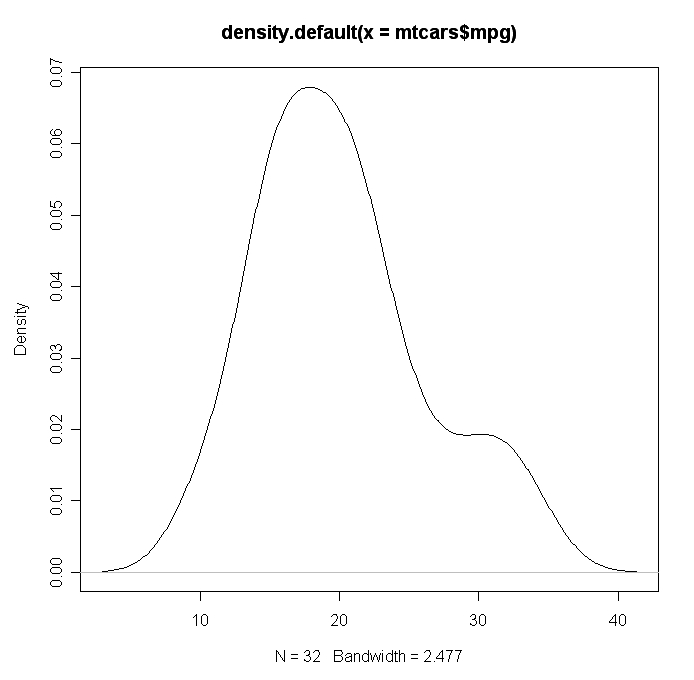dotplot dot plots(suited for smaller datasets, the crucial part is choosing the size of the dots, help to identify higher density and outliers)

histogram histograms

qqmath quantile plots against mathematical distributions: (quantile) plot is a probability plot, which is a graphical method which identifies quantiles i.e  cut points dividing the range of a probability distribution into continuous intervals with equal probabilities (visualization and interpretation in diagnostic plots)

stripplot 1-dimensional scatterplot: Strip plots are a form of scatter plots using only 1 expression.

```stripchart(airquality\$Ozone,
main="Mean ozone in parts per billion at Roosevelt Island",
xlab="Parts Per Billion",
ylab="Ozone",
method="jitter",
col="orange",
pch=1
)```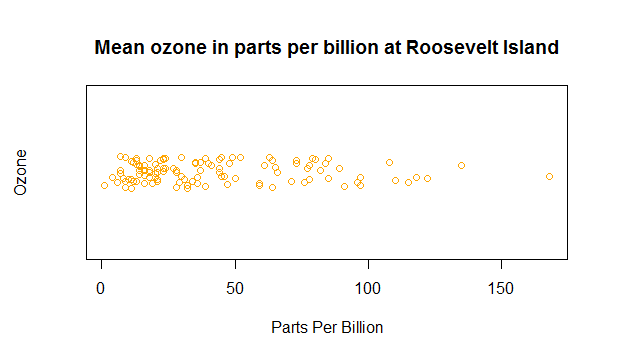Bivariate:

qq q-q plot for comparing two distributions: A q-q plot is a plot of the quantiles of the first data set against the quantiles of the second data set. By a quantile, we mean the fraction (or per cent) of points below the given value. That is, the 0.3 (or 30%) quantile is the point at which 30% of the data fall below and 70% fall above that value.

xyplot scatter plot (and possibly a lot more)

Trivariate:

levelplot level plots (similar to image plots in R): the level plot is a type of graph that is used to display a surface in two rather than three dimensions – the surface is viewed from above as if we were looking straight down and is an alternative to a contour plot – geographic data

contourplot contour plots: used to represent geographical data, a large number of values at closed intervals.

wireframe 3-D surfaces (similar to persp plots in R) Generic functions to draw 3d scatter plots and surfaces.

Hypervariate:

Splom: The scatterplot matrix, known acronymically as SPLOM, is a relatively uncommon graphical tool that uses multiple scatterplots to determine the correlation (if any) between a series of variables

rfs :residual and fitted value plot (also see oneway): It is a scatter plot of residuals on the y-axis and fitted values (estimated responses) on the x-axis. The plot is used to detect non-linearity, unequal error variances, and outliers. (interpretation in diagnostic plots)

Tmd: Tukey Mean-Difference plot: The Tukey mean-difference plot is an adaption of the quantile-quantile plot. It plots the difference of the quantiles against their average. The advantage of the Tukey mean-difference compared to the q-q plot is that it converts interpretation of the differences around a 45-degree diagonal line to the interpretation of differences around a horizontal zero line. However, the Tukey mean-difference plot should only be applied if the two variables are on a common scale.

Clustering methods are unsupervised learning also.

K-means:

In K-means clustering, we have to specify the number of clusters we want the data to be grouped into. The algorithm randomly assigns each observation to a cluster and finds the centroid of each cluster. Then, the algorithm iterates through two steps:

• Reassign data points to the cluster whose centroid is closest.
• Calculate new centroid of each cluster.

These two steps are repeated till the within-cluster variation cannot be reduced any further. The within-cluster variation is calculated as the sum of the Euclidean distance between the data points and their respective cluster centroids.

Whereas, hierarchical clustering is an alternative approach which builds a hierarchy from the bottom-up, and doesn’t require us to specify the number of clusters beforehand.

The algorithm works as follows:

• Put each data point in its own cluster.
• Identify the closest two clusters and combine them into one cluster.
• Repeat the above step till all the data points are in a single cluster.

Once this is done, it is usually represented by a dendrogram like structure.

Example: Let’s use iris dataset from the dataset library.

• Kmeans
```library(datasets)
library(ggplot2)
irisCluster <- kmeans(iris[, 3:4], 3, nstart = 20)
# nstart = 20 means R will have 20 random start and select one with lowest within cluster variation.
irisCluster ```

This gives you the cluster means, clustering vectors & wcss by cluster (implicit objective function to measure the distance of observations from their cluster centroids).

• H-clustering

We can use hclust for this. hclust requires us to provide the data in the form of a distance matrix. We can do this by using dist. By default, the complete linkage method is used.

There are a few ways to determine how close two clusters are:

• Complete linkage clustering: Find the maximum possible distance between points belonging to two different clusters.
• Single linkage clustering: Find the minimum possible distance between points belonging to two different clusters.
• Mean linkage clustering: Find all possible pairwise distances for points belonging to two different clusters and then calculate the average.
• Centroid linkage clustering: Find the centroid of each cluster and calculate the distance between centroids of two clusters.

Complete linkage and mean linkage clustering are the ones used most often.

```clusters <-  hclust(dist(iris[, 3:4]))
plot(clusters)

clusters <- hclust(dist(iris[, 3:4]), method = 'average')
plot(clusters)```

This will generate a dendrogram allowing you to figure out the best choices for the total number of clusters.

Yes, rotation (orthogonal) is necessary because it maximizes the difference between the variance captured by the component. This makes the components easier to interpret. Not to forget, that’s the motive of doing PCA where we aim to select fewer components (than features) which can explain the maximum variance in the data set. By doing rotation, the relative location of the components doesn’t change, it only changes the actual coordinates of the points.

```input = read.csv("iris.csv")
names(input)
str(input)
model = prcomp(input[,1:4], scale=TRUE)
model\$sdev
model\$rotation
model\$center
model\$scale

par(mfrow=c(2,2))
plot(model\$x[,1], col=input[,5])
plot(model\$x[,2], col=input[,5])
plot(model\$x[,3], col=input[,5])
plot(model\$x[,4], col=input[,5])```

The above code would produce scatter plots in a 2 by 2 matrix, which would indicate variance explained by the components. The more the variance explained the more robust the factors created.

Types:

• Bernoulli Distribution: A Bernoulli distribution has only two possible outcomes, namely 1 (success) and 0 (failure), and a single trial. So the random variable X which has a Bernoulli distribution can take value 1 with the probability of success, say p, and the value 0 with the probability of failure, say q or 1-p.
• Uniform Distribution: When you roll a fair die, the outcomes are 1 to 6. The probabilities of getting these outcomes are equally likely and that is the basis of a uniform distribution. Unlike Bernoulli Distribution, all the n number of possible outcomes of a uniform distribution are equally likely.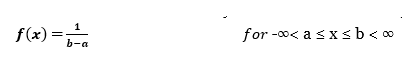• Binomial Distribution:
1. Each trial is independent.
2. There are only two possible outcomes in a trial- either a success or a failure.
3. A total number of n identical trials are conducted.
4. The probability of success and failure is the same for all trials. (Trials are identical.)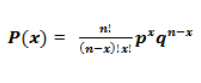• Normal Distribution: Any distribution is known as Normal distribution if it has the following characteristics:
1. The mean, median and mode of the distribution coincide.
2. The curve of the distribution is bell-shaped and symmetrical about the line x=μ.
3. The total area under the curve is 1.
4. Exactly half of the values are to the left of the centre and the other half to the right.
5. Poisson distribution:  Poisson Distribution is applicable in situations where events occur at random points of time and space wherein our interest lies only in the number of occurrences of the event. For e.g.,
6. The number of emergency calls recorded at a hospital in a day.
7. The number of thefts reported in an area on a day.
8. The number of customers arriving at a salon in an hour.
9. The number of suicides reported in a particular city.
10. The number of printing errors at each page of the book.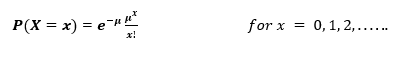• Exponential Distribution: Let’s consider the call centre example one more time. Here, the exponential distribution comes to our rescue. Exponential distribution models the interval of time between the calls.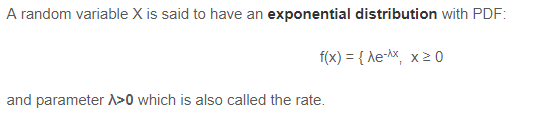It states that, if a population is normally distributed, the sample means for samples taken from the population are also normally distributed regardless of the sample size. i.e. if a sample of size n is drawn from the population for a sufficiently larger sample size ( n >= 30); then the sample means are appx. Normally distributed regardless of the shape of the population distribution.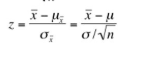Let’s denote the dependent variable by DV, and the independent variables by IV1, IV2, etc. Then the R code to get the Adjusted R-Squared is the following:

`summary(lm(DV ~ IV1 + IV2 + ..., dataset))\$adj.r.squared `
```bday = as.numeric(as.Date("2018-12-05") - as.Date("1992-07-08")) / 365
round(bday)```

loess() function helps smoothening and better fitting on smooth curves. This style interpolates lots of extra points and gets you a curve that is very smooth.

```x <- 1:10
y <- c(2,4,6,8,7,12,14,16,18,20)
lo <- loess(y~x)
plot(x,y)
lines(predict(lo), col='red', lwd=2)```

Using one hot encoding, the dimensionality (a.k.a features) in a dataset get increased because it creates a new variable for each level present in categorical variables. For example: let’s say we have a variable ‘color’. The variable has 3 levels namely Red, Blue and Green. One hot encoding ‘color’ variable will generate three new variables as Color.Red, Color.Blue and Color.Green containing 0 and 1 value. In label encoding, the levels of categorical variables get encoded as 0 and 1, so no new variable is created. Label encoding is majorly used for binary variables.

#Example:

`a) OHE – `
```library(caret)
customers <- data.frame(
id=c(10, 20, 30, 40, 50),
gender=c('male', 'female', 'female', 'male', 'female'),
outcome=c(1, 1, 0, 0, 0))

# dummify the data
dmy <- dummyVars(" ~ .", data = customers)
trsf <- data.frame(predict(dmy, newdata = customers))

OR
library(data.table)
library(mltools)
customers_1h <- one_hot(as.data.table(customers))
```
`b) Label Encoding:`
```y = data.frame(label=c("Low","High","Medium",NA,"High"))
y\$ label <- replace(y\$ label, y\$ label == "high", 0)
y\$ label <- replace(y\$ label, y\$ label == "medium", 1)
y\$ label <- replace(y\$ label, y\$ label == "low", 2)
y\$ label <- replace(y\$ label, y\$ label == "NA", 3)```

lapply- l in lapply stands for list. The output is in the form of a list,

sapply does the same job but is used to show the output in the form of vector or data frame &,

tapply is used to compute a measure (mean, median, min, max) for each factor variable in a vector.

#Example: Let’s try to measure the speed and stopping distances of the car dataset

• lapply & sapply

```dt <- cars
lmn_cars <- lapply(dt, min)
smn_cars <- sapply(dt, min)

OUTPUT
lmn_cars-
## \$speed
##  4
## \$dist
##  2

smn_cars -
## speed  dist ```
`##     4     2`
• tapply

```data(iris)
tapply(iris\$Sepal.Width, iris\$Species, median)
OUTPUT
##     setosa versicolor  virginica
##        3.4        2.8        3.0```

Since the data is spread across the median, let’s assume it’s a normal distribution. We know, in a normal distribution, 68% of the data lies in 1 standard deviation from the mean (or mode, median), which leaves 32% of the data unaffected. Therefore, 32% of the data would remain unaffected by missing values.

In an imbalanced dataset, accuracy should not be used as a measure of performance because 96% (as given) might only be predicting majority class correctly, but our class of interest is minority class (4%). Hence, in order to evaluate model performance, we should use Sensitivity (True Positive Rate), Specificity (True Negative Rate), F measure to determine the class wise performance of the classifier. If the minority class performance is found to be poor, we can undertake the following steps:

1. We can use undersampling, oversampling or SMOTE to make the data balanced.
2. We can alter the prediction threshold value by doing probability calibration and finding an optimal threshold using AUC-ROC curve.
3. We can assign weight to classes such that the minority classes get larger weight.
4. We can also use anomaly detection.

Low bias occurs when the model’s predicted values are near to actual values. In other words, the model becomes flexible enough to mimic the training data distribution. While it sounds like a great achievement, but not to forget, a flexible model has no generalization capabilities. It means, when this model is tested on unseen data, it gives disappointing results.

In such situations, we can use the bagging algorithm (like the random forest) to tackle high variance problem. Bagging algorithms divide a data set into subsets made with repeated randomized sampling. Then, these samples are used to generate a set of models using a single learning algorithm. Later, the model predictions are combined using voting (classification) or averaging (regression). Also, to combat high variance, we can:

1. Use the regularization technique, where higher model coefficients get penalized, hence lowering model complexity.
2. Use top n features from variable importance chart. Maybe, with all the variable in the data set, the algorithm is having difficulty in finding the meaningful signal.

RF is a bagging technique whereas GBM is a boosting technique. In the bagging technique, a data set is divided into n samples using randomized sampling. Then, using a single learning algorithm a model is built on all samples. Later, the resultant predictions are combined using voting or averaging. Bagging is done is parallel. In boosting, after the first round of predictions, the algorithm weighs misclassified predictions higher, such that they can be corrected in the succeeding round. This sequential process of giving higher weights to misclassified predictions continue until a stopping criterion is reached.

Random forest improves model accuracy by reducing variance (mainly). The trees grown are uncorrelated to maximize the decrease in variance. On the other hand, GBM improves accuracy by reducing both bias and variance in a model

OLS and Maximum likelihood are the methods used by the respective regression methods to approximate the unknown parameter (coefficient) value.

In simple words, Ordinary least square (OLS) is a method used in linear regression which approximates the parameters resulting in a minimum distance between actual and predicted values. Maximum Likelihood helps in choosing the values of parameters which maximizes the likelihood that the parameters are most likely to produce observed data

Multicollinearity can be detected using VIF and Tolerance, where VIF is the reciprocal of the Tolerance ( Tolerance = 1 – R^2)

VIF: Greater than 3 denotes that there is multicollinearity, however, greater than 10 shows serious multicollinearity

Whereas when checking the tolerance, the value of 0.5 or higher is generally considered acceptable.

Autocorrelation: can be tested via, Durbin Watson Test.

barlett.test( ) is used to provide a parametric k-sample test of the equality of variances. Barlett’s measure, test the null hypothesis that the original correlation matrix is an identity matrix. For factor analysis, we need some relationships between the variables and if the R- matrix were an identity matrix then all the correlation coefficients would be zero. Therefore, we want this test to be significant (i.e. have significance value less than 0.05).

A significant test will tell us that R-matrix is not an identity matrix, therefore there is some relationship between the variables we hope to include in the analysis.

Data frame can contain heterogeneous inputs while a matrix cannot. In matrix, only similar data types can be stored whereas in a data frame there can be different data types like characters, integers or other data frames.

`mean impute <- function(x) {x [is.na(x)] <- mean(x, na.rm = TRUE); x}`

Rbind function in R row binds the data frames. In other words, Rbind in R appends or combines vector, matrix or data frame by rows. Let's see an example of row bind in R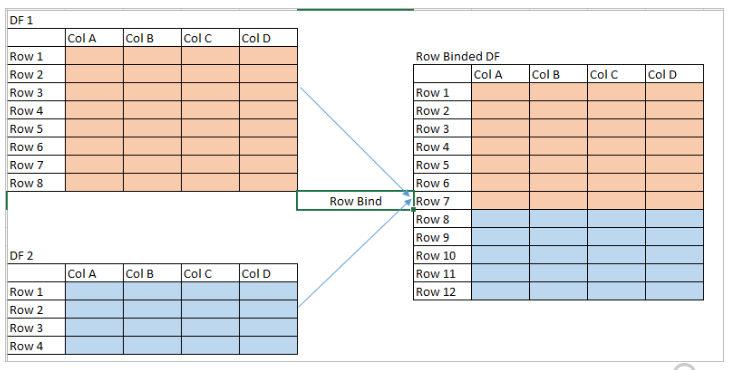Cbind  - Column Bind in R appends or combines vector, matrix or data frame by columns. Lets see column bind in R with an example.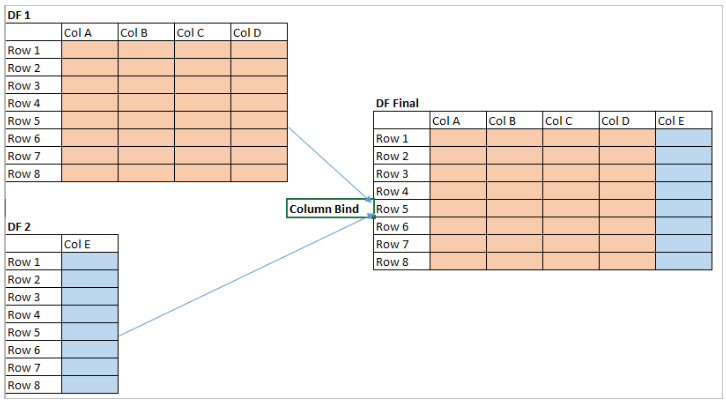The function t () is used for transposing a matrix.

#Example –

`Use t (m), where m is a matrix. `

Through k-Fold Cross Validation, we need to estimate the "unbiased error" on the dataset from this model. The mean is a good estimate of how the model will perform on the dataset. Then once we are convinced that the average error is acceptable, we train the same model on all of the dataset.

#Example: Below is an implementation of K-fold cross validation using R

```# k-Fold Cross Validation
# Importing the dataset
dataset = dataset[3:5]
# Encoding the target feature as factor
dataset\$Purchased = factor(dataset\$Purchased, levels = c(0, 1))
# Splitting the dataset into the Training set and Test set
library(caTools) # install.packages('caTools')
set.seed(123)
split = sample.split(dataset\$Purchased, SplitRatio = 0.75)
training_set = subset(dataset, split == TRUE)
test_set = subset(dataset, split == FALSE)
# Feature Scaling
training_set[-3] = scale(training_set[-3])
test_set[-3] = scale(test_set[-3])
# Fitting Kernel SVM to the Training set
# install.packages('e1071')
library(e1071)
classifier = svm(formula = Purchased ~ .,
data = training_set,
type = 'C-classification',
# Applying k-Fold Cross Validation
# install.packages('caret')
library(caret)
folds = createFolds(training_set\$Purchased, k = 10)
cv = lapply(folds, function(x) {
training_fold = training_set[-x, ],
test_fold = training_set[x, ],
classifier = svm(formula = Purchased ~ .,
data = training_fold,
type = 'C-classification',
y_pred = predict(classifier, newdata = test_fold[-3])
cm = table(test_fold[, 3], y_pred)
accuracy = (cm[1,1] + cm[2,2]) / (cm[1,1] + cm[2,2] + cm[1,2] + cm[2,1])
return(accuracy)
})
accuracy = mean(as.numeric(cv)) #Accuracy```

We can find a relevant number of the tree through experimentation and manual tweaking. We can use enough trees to get a good accuracy, but shouldn’t use too many trees because that
could cause overfitting. Also, to find out the optimal number of trees, we can apply model selection, which is done with a technique called Parameter Tuning (Grid Search with k-Fold Cross Validation).

#Example:

```# Importing the dataset
dataset = dataset[3:5]

# Encoding the target feature as factor

dataset\$Purchased = factor(dataset\$Purchased, levels = c(0, 1))

# Splitting the dataset into the Training set and Test set
# install.packages('caTools')
library(caTools)
set.seed(123)
split = sample.split(dataset\$Purchased, SplitRatio = 0.75)
training_set = subset(dataset, split == TRUE)
test_set = subset(dataset, split == FALSE)

# Feature Scaling
training_set[-3] = scale(training_set[-3])
test_set[-3] = scale(test_set[-3])

# Fitting Kernel SVM to the Training set
# install.packages('e1071')
library(e1071)
classifier = svm(formula = Purchased ~ .,
data = training_set,
type = 'C-classification',

# Predicting the Test set results
y_pred = predict(classifier, newdata = test_set[-3])

# Making the Confusion Matrix
cm = table(test_set[, 3], y_pred)

# Applying k-Fold Cross Validation
# install.packages('caret')
library(caret)
folds = createFolds(training_set\$Purchased, k = 10)
cv = lapply(folds, function(x) {
training_fold = training_set[-x, ]
test_fold = training_set[x, ]
classifier = svm(formula = Purchased ~ .,
data = training_fold,
type = 'C-classification',
y_pred = predict(classifier, newdata = test_fold[-3])
cm = table(test_fold[, 3], y_pred)
accuracy = (cm[1,1] + cm[2,2]) / (cm[1,1] + cm[2,2] + cm[1,2] + cm[2,1])
return(accuracy)
})
accuracy = mean(as.numeric(cv))

# Applying Grid Search to find the best parameters
# install.packages('caret')
library(caret)
classifier = train(form = Purchased ~ ., data = training_set, method = 'svmRadial')
classifier
classifier\$bestTune```

Output : Tuning parameter 'sigma' was held constant at a value of 2.251496. Accuracy was used to select the optimal model using the largest value. The final values used for the model were sigma = 2.251496 and C = 1.

MANOVA stands for multivariate analysis of variance. By using MANOVA we can test more than one dependent variable simultaneously i.e. the technique examines the relationship between the several categorical variables and two or more metric dependent variables.

However, the sample size is an issue, with 15-20 observations needed per cell. However, too many observations per cell (over 30) and the technique loses its practical significance.

Analysis of variance (ANOVA) assesses the difference between the groups ( by using T-test for 2 means and F test for 3 or more means) whereas MANOVA examines the dependent relationship between a set of dependent measures across a set of groups.

We can check if a model works well for data in many different ways. We pay great attention to regression results, such as slope coefficients, p-values, or R2 that tell us how well a model represents given data. That’s not the whole picture though.

An alternate approach could be to check Residuals as they could show how poorly a model represents data. Residuals are leftover of the outcome variable after fitting a model (predictors) to data and they could reveal unexplained patterns in the data by the fitted model. Using this information, not only could you check if linear regression assumptions are met, but you could improve your model in an exploratory way.

Diagnostic plots hence is used to check the normality of the residuals, heteroscedasticity and other influential observations.

There are four types of diagnostic plots:

• Residuals vs Fitted

This plot shows if residuals have non-linear patterns. There could be a non-linear relationship between predictor variables and an outcome variable and the pattern could show up in this plot if the model doesn’t capture the non-linear relationship. If you find equally spread residuals around a horizontal line without distinct patterns, that is a good indication you don’t have non-linear relationships.

Let’s look at residual plots from a ‘good’ model and a ‘bad’ model. The good model data are simulated in a way that meets the regression assumptions very well, while the bad model data are not.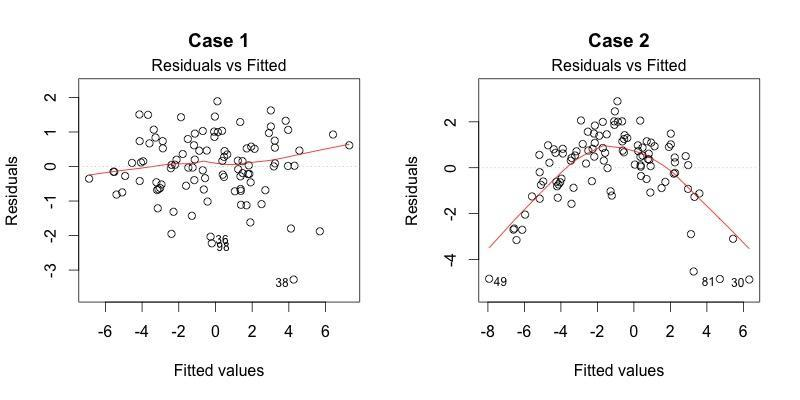We can’t see any distinctive pattern in Case 1, but a parabola in Case 2, where the non-linear relationship was not explained by the model and was left out in the residuals.

•  Normal Q-Q

This plot shows if residuals are normally distributed.

We check if the residuals follow a straight line well or do they deviate severely? It’s good if residuals are lined well on the straight dashed line.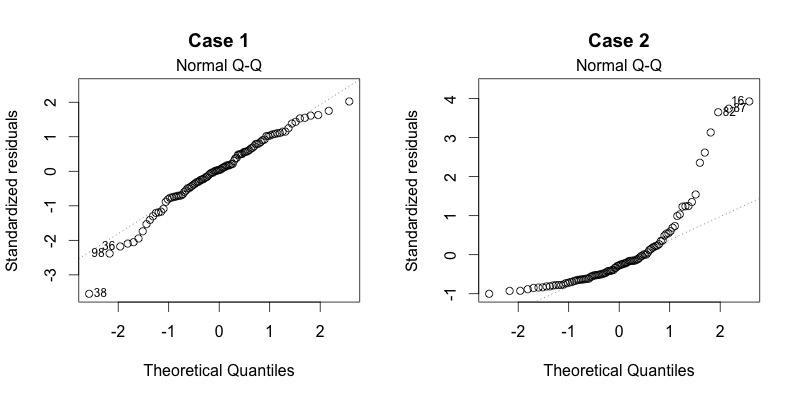•  Scale-Location

It’s also called Spread-Location plot. This plot shows if residuals are spread equally along the ranges of predictors. This is how you can check the assumption of equal variance (homoscedasticity). It’s good if you see a horizontal line with equally (randomly) spread points.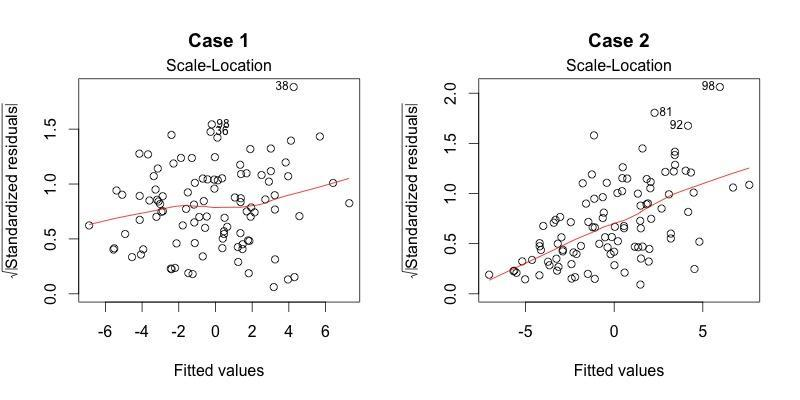In Case 1, the residuals appear randomly spread. Whereas, in Case 2, the residuals begin to spread wider along the x-axis as it passes around 5. Because the residuals spread wider and wider, the red smooth line is not horizontal and shows a steep angle in Case 2.

•  Residuals vs Leverage

This plot helps us to find influential cases (i.e., subjects) if any. Not all outliers are influential in linear regression analysis (whatever outliers mean). Even though data have extreme values, they might not be influential to determine a regression line. That means the results wouldn’t be much different if we either include or exclude them from the analysis. They follow the trend in the majority of cases and they don’t really matter; they are not influential. On the other hand, some cases could be very influential even if they look to be within a reasonable range of values. They could be extreme cases against a regression line and can alter the results if we exclude them from the analysis. Another way to put it is that they don’t get along with the trend in the majority of the cases.

Unlike the other plots, this time patterns are not relevant. We watch out for outlying values at the upper right corner or at the lower right corner. Those spots are the places where cases can be influential against a regression line.

Survival analysis deals with predicting the time when a specific event is going to occur. It is also known as failure time analysis or analysis of time to death. For example, predicting the number of days a person with cancer will survive or predicting the time when a mechanical system is going to fail.

The R package named survival is used to carry out survival analysis. This package contains the function Surv() which takes the input data as a R formula.

# Example: Below is an implementation of Survival analysis in R on the dataset named ‘pbc’ present in survival package.

```install.packages("survival")
library("survival")
# Print first few rows.